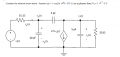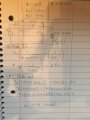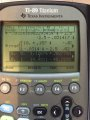# Finding the voltage/vector phasor (Mistake in my wrong solution)

#### libnitz

Joined Jan 29, 2016
17
Hi,I have being asked to calculate the phase voltage across the capacitor 5pF vo(t)

Here is my solution:I used my TI-89 to solve the system of equations:What I did:
I tried using node analysis to find vo(t) by labeling V1 and V2. I combined the resistor and capacitor at the right end of the circuit to 1 single device with a combined impedance of 14.42 - 22.65i. I then worked out 2 equations:

Edit:
Node 2
-0.0314i V1 +(0.02+0.0628i)V2 =2.5V1
Node 1
(-0.0314i - 2.5i) V1 + (0.02+0.0628i) V2 = 0

My answer for V2 is 14.48 - 40.22i but I'm wrongNeed help. any is appreciated.

Edit: I mislabeled my Nodes in the pic I took of my solns; Node 1 is supposed to be Node 2 and vice versa. The derivation of my nodes still looks correct to me.

Last edited: